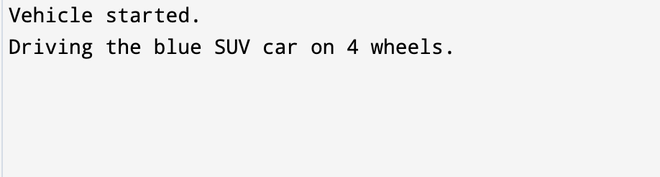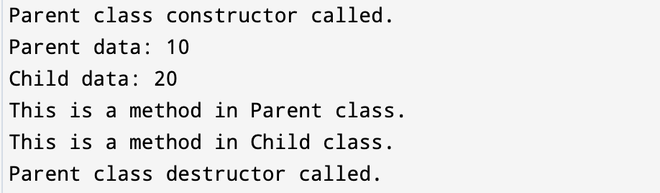Open In App

# What all is inherited from parent class in C++?

Following are the things that a derived class inherits from its parent:

• All the public and protected data members and member functions of the base class. Private members are not inherited.
• The assignment operator (=) of the base class.
• The type of the base class, which allows the derived class to be treated as a base class object when necessary.

Following are the properties which a derived class doesn’t inherit from its parent class:

• Constructors and destructor: The constructors and destructor of a base class are not inherited by a derived class. However, the constructor of the base class can be invoked explicitly from the derived class constructor using the initialization list.
• Friend functions: The friend functions of a base class are not inherited by a derived class. Friend functions are those functions that have access to the private and protected members of a class.
• Overloaded operators: The overloaded operators of a base class are not inherited by a derived class. Overloaded operators are those operators that are redefined to perform specific operations on the objects of a class. However, a derived class can overload the operators that are inherited from its parent class.

Example 1: In this example, we will see how the derived class inherits from its parent.

## C++

 `#include ``using` `namespace` `std;` `// Parent class``class` `Vehicle {``public``:``    ``int` `wheels;``    ``string color;``    ``void` `start() { cout << ``"Vehicle started."` `<< endl; }``};` `// Child class``class` `Car : ``public` `Vehicle {``public``:``    ``string model;``    ``void` `drive()``    ``{``        ``cout << ``"Driving the "` `<< color << ``" "` `<< model``             ``<< ``" car on "` `<< wheels << ``" wheels."` `<< endl;``    ``}``};` `int` `main()``{``    ``// Create an object of the child class``    ``Car myCar;``    ``myCar.wheels = 4;``    ``myCar.color = ``"blue"``;``    ``myCar.model = ``"SUV"``;` `    ``// Call functions from the parent and child classes``    ``myCar.start();``    ``myCar.drive();` `    ``return` `0;``}`Example 2: demonstration of the properties that a derived class doesn’t inherit from its parent class.

## C++

 `#include ``using` `namespace` `std;` `class` `Parent {``public``:``    ``int` `parent_data;``    ``void` `parent_method()``    ``{``        ``cout << ``"This is a method in Parent class."` `<< endl;``    ``}``    ``Parent()``    ``{``        ``cout << ``"Parent class constructor called."` `<< endl;``    ``}``    ``~Parent()``    ``{``        ``cout << ``"Parent class destructor called."` `<< endl;``    ``}``};` `class` `Child : ``public` `Parent {``public``:``    ``int` `child_data;``    ``void` `child_method()``    ``{``        ``cout << ``"This is a method in Child class."` `<< endl;``    ``}``};` `int` `main()``{``    ``Child c;``    ``c.parent_data = 10;``    ``c.child_data = 20;``    ``cout << ``"Parent data: "` `<< c.parent_data << endl;``    ``cout << ``"Child data: "` `<< c.child_data << endl;``    ``c.parent_method();``    ``c.child_method();``    ``return` `0;``}`

Output:Explanation: In this example, the Child class is derived from the Parent class. The Child class inherits the parent_data member and parent_method() function from the Parent class. However, the Child does not inherit the Parent class constructor, destructor, and friend functions.